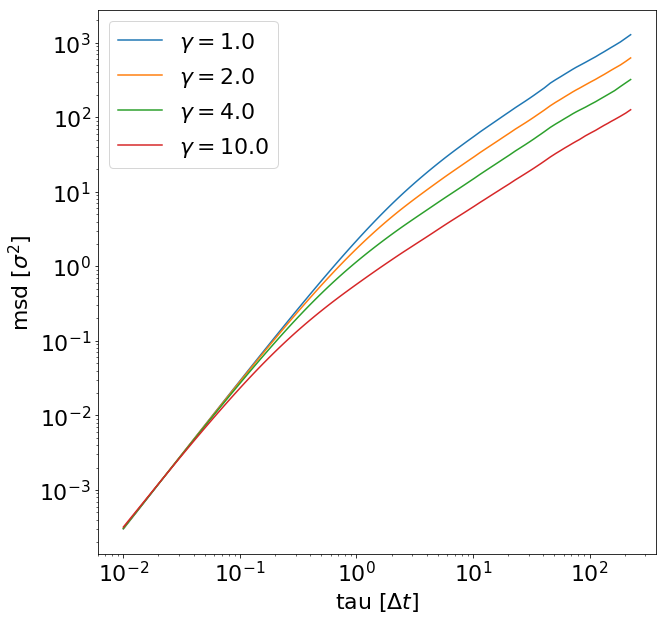# Tutorial 4 : The lattice-Boltzmann method in ESPResSo - Part 2¶

## 5 Polymer Diffusion¶

In these exercises we want to use the LBM-MD-Hybrid to reproduce a classic result of polymer physics: the dependence of the diffusion coefficient of a polymer on its chain length. If no hydrodynamic interactions are present, one expects a scaling law $D \propto N ^{- 1}$ and if they are present, a scaling law $D \propto N^{- \nu}$ is expected. Here $\nu$ is the Flory exponent that plays a very prominent role in polymer physics. It has a value of $\sim 3/5$ in good solvent conditions in 3D. Discussions on these scaling laws can be found in polymer physics textbooks like [4–6].

The reason for the different scaling law is the following: when being transported, every monomer creates a flow field that follows the direction of its motion. This flow field makes it easier for other monomers to follow its motion. This makes a polymer (given it is sufficiently long) diffuse more like a compact object including the fluid inside it, although it does not have clear boundaries. It can be shown that its motion can be described by its hydrodynamic radius. It is defined as:

\begin{equation} \left\langle \frac{1}{R_h} \right\rangle = \left\langle \frac{1}{N^2}\sum_{i\neq j} \frac{1}{\left| r_i - r_j \right|} \right\rangle \end{equation}

This hydrodynamic radius exhibits the scaling law $R_h \propto N^{\nu}$ and the diffusion coefficient of a long polymer is proportional to its inverse $R_h$.

For shorter polymers there is a transition region. It can be described by the Kirkwood-Zimm model:

\begin{equation} D=\frac{D_0}{N} + \frac{k_B T}{6 \pi \eta } \left\langle \frac{1}{R_h} \right\rangle \end{equation}

Here $D_0$ is the monomer diffusion coefficient and $\eta$ the viscosity of the fluid. For a finite system size the second part of the diffusion is subject of a $1/L$ finite size effect, because hydrodynamic interactions are proportional to the inverse distance and thus long ranged. It can be taken into account by a correction:

\begin{equation} D=\frac{D_0}{N} + \frac{k_B T}{6 \pi \eta } \left\langle \frac{1}{R_h} \right\rangle \left( 1- \left\langle\frac{R_h}{L} \right\rangle \right) \end{equation}

It is quite difficult to prove this formula computationally with good accuracy. It will need quite some computational effort and a careful analysis. So please don't be too disappointed if you don't manage to do so.

We want to determine the long-time self diffusion coefficient from the mean square displacement of the center-of-mass a single polymer. For large $t$ the mean square displacement is proportional to the time and the diffusion coefficient occurs as a prefactor:

\begin{equation} D = \lim_{t\to\infty}\left[ \frac{1}{6t} \left\langle \left(\vec{r}(t) - \vec{r}(0)\right)^2 \right\rangle \right]. \end{equation}

This equation can be found in virtually any simulation textbook, like . We will therefore set up a polymer in an LB fluid, simulate for an appropriate amount of time, calculate the mean square displacement as a function of time and obtain the diffusion coefficient from a linear fit. However we will have a couple of steps inbetween and divide the full problem into subproblems that allow to (hopefully) fully understand the process.

### 5.1 Step 1: Diffusion of a single particle¶

Our first step is to investigate the diffusion of a single particle that is coupled to an LB fluid by the point coupling method:

In :
import logging
import sys

import espressomd
import espressomd.accumulators
import espressomd.lb
import espressomd.observables

logging.basicConfig(level=logging.INFO, stream=sys.stdout)

# Constants
LOOPS = 40000
STEPS = 10

# System setup
system = espressomd.System(box_l= * 3)
system.time_step = 0.01
system.cell_system.skin = 0.4

lbf = espressomd.lb.LBFluidGPU(kT=1, seed=132, agrid=1, dens=1, visc=5, tau=0.01)

# Setup observable
pos = espressomd.observables.ParticlePositions(ids=(0,))

# Run for different friction constants
lb_gammas = [1.0, 2.0, 4.0, 10.0]
msd_results = []

for gamma in lb_gammas:
system.auto_update_accumulators.clear()
system.thermostat.turn_off()
system.thermostat.set_lb(LB_fluid=lbf, seed=123, gamma=gamma)
# Equilibrate
logging.info("Equilibrating the system.")
system.integrator.run(1000)
logging.info("Equilibration finished.")
# Setup observable correlator
correlator = espressomd.accumulators.Correlator(obs1=pos, tau_lin=16, tau_max=LOOPS * STEPS, delta_N=1,

logging.info("Sampling started for gamma = {}.".format(gamma))
for i in range(LOOPS):
system.integrator.run(STEPS)
correlator.finalize()
msd_results.append(correlator.result())

logging.info("Sampling finished.")

INFO:root:Equilibrating the system.
INFO:root:Equilibration finished.
INFO:root:Sampling started for gamma = 1.0.
INFO:root:Equilibrating the system.
INFO:root:Equilibration finished.
INFO:root:Sampling started for gamma = 2.0.
INFO:root:Equilibrating the system.
INFO:root:Equilibration finished.
INFO:root:Sampling started for gamma = 4.0.
INFO:root:Equilibrating the system.
INFO:root:Equilibration finished.
INFO:root:Sampling started for gamma = 10.0.
INFO:root:Sampling finished.


#### Plotting the results¶

In :
%matplotlib inline
import matplotlib.pyplot as plt

In :
plt.rcParams.update({'font.size': 22})

plt.figure(figsize=(10,10))
plt.xlabel('tau [$\Delta t$]')
plt.ylabel('msd [$\sigma^2$]')
for index, r in enumerate(msd_results):
# We skip the first entry since it's zero by definition and cannot be displayed
# in a loglog plot. Furthermore, we only look at the first 100 entries due to
# lack of good statistics for larger times.
msd = r[1:100, 2] + r[1:100, 3] + r[1:100, 4]
plt.loglog(r[1:100, 0], msd, label=r"$\gamma=${}".format(str(lb_gammas[index])))
plt.legend()
plt.show()#### Calculating the diffusion coefficient¶

In this script an LB fluid and a single particle are created and thermalized. The random forces on the particle and within the LB fluid will cause the particle to move. The mean squared displacement is calculated during the simulation via a multiple-tau correlator. Can you give an explanation for the quadratic time dependency for short times? Use the function curve_fit from the module scipy.optimize to produce a fit for the linear regime and determine the diffusion coefficients for the different $\gamma$s. Calculate the diffusion coefficient for all cases and plot them as a function of γ. What relation do you observe?

 S. Succi. The lattice Boltzmann equation for fluid dynamics and beyond. Clarendon Press, Oxford, 2001.
 B. Dünweg and A. J. C. Ladd. Advanced Computer Simulation Approaches for Soft Matter Sciences III, chapter II, pages 89–166. Springer, 2009.
 B. Dünweg, U. Schiller, and A.J.C. Ladd. Statistical mechanics of the fluctuating lattice-Boltzmann equation. Phys. Rev. E, 76:36704, 2007.
 P. G. de Gennes. Scaling Concepts in Polymer Physics. Cornell University Press, Ithaca, NY, 1979.
 M. Doi. Introduction to Polymer Physics. Clarendon Press, Oxford, 1996.
 Michael Rubinstein and Ralph H. Colby. Polymer Physics. Oxford University Press, Oxford, UK, 2003.
 Daan Frenkel and Berend Smit. Understanding Molecular Simulation. Academic Press, San Diego, second edition, 2002.